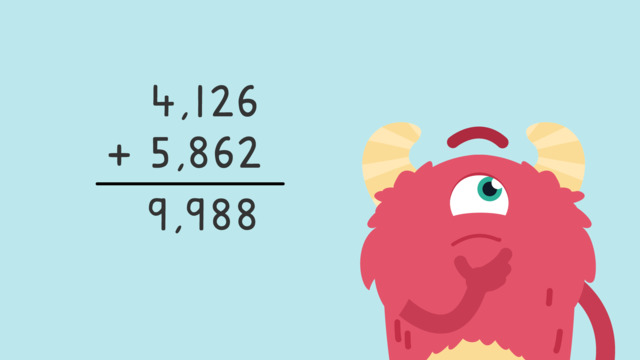# Adding Larger Numbers — Let's Practice!Rating

Ø 5.0 / 1 ratings
The authorsTeam Digital
Adding Larger Numbers — Let's Practice!
CCSS.MATH.CONTENT.4.NBT.B.4

## Basics on the topicAdding Larger Numbers — Let's Practice!

Today we are practicing adding larger numbers with Razzi! This video contains examples to help you further practice and grow confident in this area.

## Adding Larger Numbers — Let's Practice! exercise

Would you like to apply the knowledge you’ve learned? You can review and practice it with the tasks for the video Adding Larger Numbers — Let's Practice! .
• ### Add these three digit numbers together.

Hints

Remember, add the ones place first, then the tens place, then the hundreds place, and lastly, the thousands place.

There is no regrouping in this problem.

Solution

Adding the ones place: 6 + 1, which is 7.

Adding the tens place: 70 + 0, which is 70.

Adding the hundreds place: 400 + 100, which is 500.

Adding the thousands place: 3,000 + 6,000, which is 9,000.

These numbers together are: 9,577.

• ### Add the three digit numbers with regrouping.

Hints

We add the ones place first. 7 + 4 = 11. 11 needs to be regrouped into the tens place.

Look at how to regroup 11. There is one ten (11), and one one (11). Remember to add this regrouped ten into the tens place.

Remember to add the comma after the thousands place. Example: six thousand two hundred forty-one would be: 6,241.

Solution

Add the ones place: 7 + 4 = 11. Regroup the 11 to one ten, and one one. The ones place is now 1.

Add the tens place: 40 + 10, + the 10 we carried. 40 + 10 + 10 = 60.

Add the hundreds place: 300 + 0 = 300.

Add the thousands place: 4,000 + 3,000 = 7,000.

The number is 7,361.

• ### Regroup as needed to add the numbers.

Hints

Remember to carry the 1 to the top of the next place value when regrouping.

Add the ones place first, then add the tens place. Regroup as needed.

Don't forget to add the comma after the thousands place.

Example: 20,567.

Solution

Add the ones place: 4 + 1 = 5.

Add the tens place: 40 + 60 = 100. We regroup 1 hundred to the hundreds place. The tens place is left with 0.

Add the hundreds place: 100 (regrouped) + 700 + 500 = 1,300. We regroup 1 1,000 to the thousands place. The hundreds place is left with 300.

Add the thousands place: 1,000 (regrouped) + 9,000 + 6,000 = 16,000.

The sum of the numbers is: 16,305.

• ### Add the two numbers by regrouping.

Hints

Remember to start with the ones place and carry the 1 to the top of the next place value when regrouping.

Don't forget to add the comma (,) after the thousands place.

Solution

Add the ones place: 1 + 1= 2.

Add the tens place: 70 + 80 = 150. Regroup the 100, the tens place is left with 50.

Add the hundreds place: 100 (regrouped) + 400 + 500 = 1,000. Regroup the 1,000 to the thousands place, the hundreds place is left with 0.

Add the thousands place: 1,000 (regrouped) + 20,000 + 3,000 = 24,000.

The number is 24,052.

• ### Add the large numbers.

Hints

Remember, start adding from the ones place.

If there is no regrouping, continue adding the tens place, hundreds place, and thousands place.

Solution

Add the ones place: 3 + 3 = 6.

Add the tens place: 90 + 0 = 90.

Add the hundreds place: 500 + 300 = 800.

Add the thousands place: 8,000 + 4,000 = 12,000.

The total is 12,896.

• ### Add the numbers by regrouping.

Hints

Remember to start with the ones place, and carry the 1 to the top of the next place value when regrouping.

Remember, add the comma (,) after the thousands place.

Example: 75,230.

Solution

Add the ones place: 9 + 9 = 18. Regroup the 10 to the tens place, the ones place is left with 8.

Add the tens place: 10 (regrouped) + 30 + 70 = 110. Regroup the 100 to the hundreds place, the tens place is left with 10.

Add the hundreds place: 100 (regrouped) + 900 + 500 = 1,500. Regroup the 1,000 to the thousands place, the hundreds place is left with 500.

Add the thousands place: 1,000 (regrouped) + 10,000 + 3,000 = 14,000.

The number is 14,518.